# Texas Go Math Grade 3 Lesson 2.2 Answer Key Unit Fractions of a Whole

Refer to our Texas Go Math Grade 3 Answer Key Pdf to score good marks in the exams. Test yourself by practicing the problems from Texas Go Math Grade 3 Lesson 2.2 Answer Key Unit Fractions of a Whole.

## Texas Go Math Grade 3 Lesson 2.2 Answer Key Unit Fractions of a Whole

Essential Question
What do the top and bottom numbers of a fraction tell?
A fraction is a number that mentions part of a whole or part of a group.
In a fraction, the top number tells how many equal numbers of parts are being counted.
The bottom number mentions how many equal parts are in the whole or in the group.

Unlock the Problem

Example 1.
Find part of a whole.
Luke’s family picked strawberries to make a strawberry pie. They cut the pie into 6 equal pieces. Luke ate 1 piece. What fraction of the strawberry pie did he eat?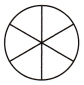Shade 1 of the 6 equal parts.
Read: one sixth Write: $$\frac{1}{6}$$
So, Luke ate ___ of the strawberry pie.
Luke ate $$\frac{1}{6}$$ of the strawberry pie.

Explanation: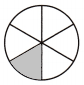Example 2.
Find part of a group.
Jake has a collection of marbles. What fraction of his collection is yellow?So, __ of Jake’s collection is yellow.
$$\frac{1}{6}$$ of Jake’s collection is yellow.

Share and Show

Math Talk
Mathematical Processes
Explain how you knew what number to write as the bottom number of the fraction in Exercise 1.
I counted the total number of equal parts in the whole. Since there are 3 equal parts, I wrote 3 as the bottom number.

Share and Show

Question 1.
What fraction names the shaded part?
Think: 1 out of 3 equal parts is shaded.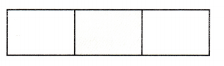$$\frac{1}{3}$$

Explanation: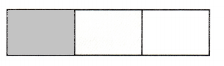1 out of 3 equal parts is shaded. Hence the fraction is $$\frac{1}{3}$$

Write the number of equal parts in the whole. Then write the fraction that names the shaded part.

Question 2.3 equal parts
$$\frac{1}{6}$$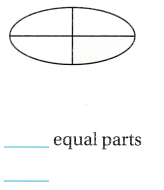4 equal parts
$$\frac{1}{4}$$

Question 4.8 equal parts
$$\frac{1}{8}$$

Write a fraction to name the yellow part of each group.

Question 5.$$\frac{1}{3}$$

Question 6.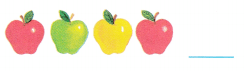$$\frac{1}{4}$$

Question 7.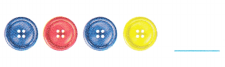$$\frac{1}{4}$$

Question 8.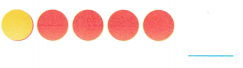$$\frac{1}{5}$$

Problem Solving

Draw a picture of the whole.

Question 9.
$$\frac{1}{2}$$ is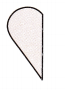$$\frac{1}{6}$$ is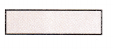Problem Solving

Use the pictures for 11—13.

Question 11.
Use Diagrams The missing parts of the pictures show what Kylie and Dylan ate for lunch. What fraction of
the pizza did Dylan eat?
$$\frac{1}{8}$$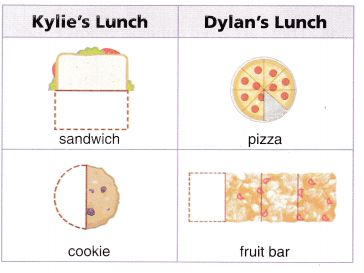Question 12.
What fraction of the cookie did Kylie eat? Write the fraction in numbers and in words.
$$\frac{1}{2}$$
one half

Question 13.
Write Math What’s the Question? The answer is $$\frac{\hat{1}}{4}$$.
What fraction of the fruit bar did Dylan eat?

Question 14.
H.O.T. Diego drew lines to divide the square into 6 pieces as shown. Then he shaded part of the square. Diego says he shaded $$\frac{\hat{1}}{6}$$ of the square. Is he correct? Explain how you know.No

Explanation:
From the given question we can tell that the square is not divided into six equal parts, so he did not shade $$\frac{1}{2}$$.

Multi-Step Riley and Chad each have a granola bar broken into equal pieces. They each eat one piece, or $$\frac{\hat{1}}{4}$$, of their granola bar. How many more pieces altogether do Riley and Chad need to eat to finish both granola bars? Draw a picture below to justify your answer.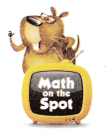6 more pieces

Explanation: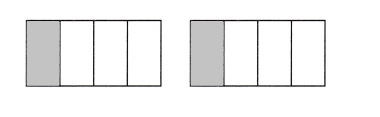Fill in the bubble for the correct answer choice.

Question 16.
There are 2 red cubes, 3 yellow cubes, and 1 blue cube in a bag. What fraction of the cubes are blue?
(A) $$\frac{1}{4}$$
(B) $$\frac{1}{3}$$
(C) $$\frac{1}{6}$$
(D) $$\frac{1}{2}$$
$$\frac{1}{6}$$

Explanation: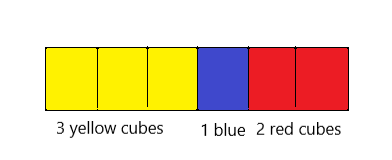There are 2 red cubes, 3 yellow cubes, and 1 blue cube in a bag. Now we need to find the fraction of the cubes that are blue. Therefore $$\frac{1}{6}$$ fraction of the cubes are blue.

Question 17.
Representations Brandon shared a large burrito with two of his friends. The missing part of the picture shows what Brandon ate. What fraction of the burrito did Brandon eat?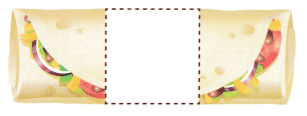(A) $$\frac{1}{4}$$
(B) $$\frac{1}{3}$$
(C) $$\frac{1}{2}$$
(D) $$\frac{1}{6}$$
$$\frac{1}{3}$$.

Explanation:
Brandon shared a large burrito with two of his friends. The fraction of the burrito did Brandon eat is $$\frac{1}{3}$$.

Question 18.
Multi-Step For an art project, Tonya and Sahil each have a piece of fabric cut into equal pieces. They each used one piece, or $$\frac{1}{3}$$ of their fabric. How many more pieces altogether do Tonya and Sahil have left to use?
(A) 2
(B) 6
(C) 4
(D) 5
4

Explanation:
Tonya and Sahil have left to use 4 more pieces altogether.

Texas Test Prep

Question 19.
Mary shaded part of a rectangle. What fraction names the part she shaded?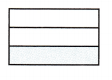(A) $$\frac{3}{1}$$
(B) $$\frac{1}{3}$$
(C) $$\frac{1}{4}$$
(D) $$\frac{1}{2}$$
$$\frac{1}{3}$$

Explanation:
As mary shaded part of a rectangle. The $$\frac{1}{3}$$ fraction names the part she shaded.

### Texas Go Math Grade 3 Lesson 2.2 Homework and Practice Answer Key

Write the number of equal parts in the whole.
Then write the fraction that names the shaded part.

Question 1.2 equal parts

Explanation:
The fraction that names the shaded part is 2 equal parts.6 equal parts

Explanation:
The fraction that names the shaded part is 6 equal parts

Question 3.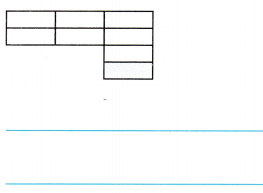8 equal parts

Explanation:
The fraction that names the shaded part is 8 equal parts

Question 4.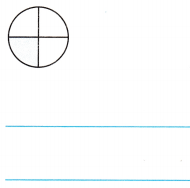4 equal parts.

Explanation:
The fraction that names the shaded part is 4 equal parts

Problem Solving

Question 5.
Toni’s fruit bar is divided into three equal pieces. Toni ate one piece. What fraction of the fruit bar did Toni eat? Draw a picture to show your answer.
$$\frac{1}{3}$$

Explanation:I have drawn a picture that is divided into three pieces and Toni ate one piece from three pieces.

The missing part of the picture shows what Kylie ate for lunch. What fraction of the sandwich did Kylie eat?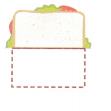$$\frac{1}{2}$$

Explanation:
Kylie ate half of the sandwich for lunch. The $$\frac{1}{2}$$ fraction of the sandwich Kylie ate.

Texas Test Prep

Lesson Check

Question 7.
The drawing below shows what the moon looks like tonight. What fraction names the shaded part?(A) $$\frac{2}{1}$$
(B) $$\frac{1}{3}$$
(C) $$\frac{1}{2}$$
(D) $$\frac{1}{6}$$
$$\frac{1}{2}$$

Explanation:
The $$\frac{1}{2}$$ fraction names the shaded part.

Question 8.
Mike divided a piece of paper into 4 equal parts. He shaded one of the parts. What fraction of the piece of(A) $$\frac{1}{2}$$
(B) $$\frac{1}{4}$$
(C) $$\frac{1}{8}$$
(D) $$\frac{4}{1}$$
$$\frac{1}{4}$$

Explanation:
Mike divided a piece of paper into 4 equal parts and shaded one of the parts. Now the $$\frac{1}{4}$$ of the piece of paper did Mike shade.

Question 9.
What is $$\frac{1}{3}$$ of this rectangle?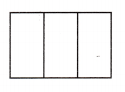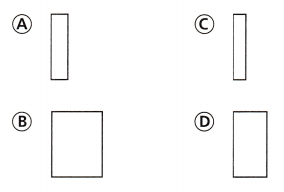Option D

Explanation:
The $$\frac{1}{3}$$ of this rectangle is option D.

Question 10.
Multi-Step Two brothers each have a sandwich divided into 4 equal pieces. Each brother eats one part, or $$\frac{1}{4}$$, of his sandwich. How many parts of the sandwiches are left altogether?
(A) 1 part
(B) 4 parts
(C) 6 parts
(D) 8 parts
6 parts

Explanation:
Two brothers each have a sandwich divided into 4 equal pieces. They both have eight pieces in total. Each one eats one part from eight pieces. Hence there are 6 parts of the sandwiches left altogether.

Multi-Step Taylor has a yellow block of cheese and an orange block of cheese. He cuts each block into eight equal parts and takes one part, or $$\frac{1}{8}$$, of each block. How many parts of the blocks of cheese are left altogether?